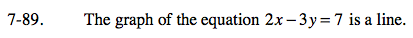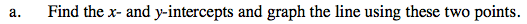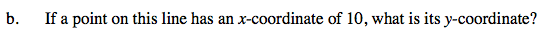### Home > CAAC > Chapter 7 > Lesson 7.3.1 > Problem7-89

7-89.Substitute x = 10 into the original equation and solve for y.

$\text{If }x =10, \ y = \frac{13}{3}.$